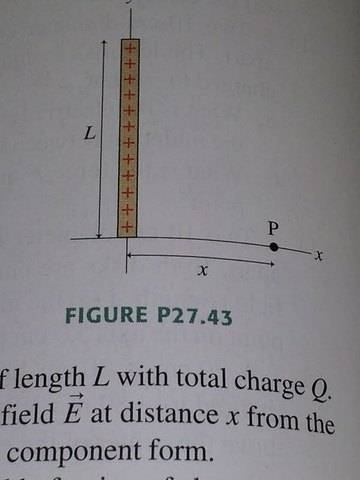# Expression of an electric field

joe_cool2

## Homework Statement

FIGURE P27.43 shows a thin rod of length L with total charge Q. Find an expression for the electric field at distance x from the rod. Give your answer in component form.## Homework Equations

Equation for an electric field:

E= $$\frac{KQ}{r^2}$$

## The Attempt at a Solution

Exi = $$\frac {1}{4\pi\epsilon_0} \frac{Q}{r^2} cos\theta$$

Ex = $$\frac{Q}{4\pi\epsilon_0} \sum_{i=1}^n \frac{1}{x^2+y^2} \frac{x}{\sqrt{x^2+y^2}}$$

Let's take the limit as n approaches infinity, thereby getting us an integral...

$$\int \frac{x}{(x^2 + y^2)^\frac{3}{2}} dy$$

$$x \int \frac{dy}{(x^2 + y^2)^\frac{3}{2}}$$

$$\frac{x^2}{x^3} \int \frac{dy}{(x^2 + y^2)^\frac{3}{2}}$$

$$\frac{sin\theta}{x} + C$$

$$\frac{y}{x\sqrt{x^2+y^2}} + C$$

The actual x-component of the electric field should be this indefinite integral evaluated from 0 to L and multiplied by Q over 4 times pi times epsilon nought...

Ex = $$\frac {Q}{4\pi\epsilon_0} \frac {L}{x\sqrt{x^2+L^2}}$$

The y-component may be evaluated in a similar manner.

Ey = $$\frac{Q}{4\pi\epsilon_0} \sum_{i=1}^n \frac{1}{x^2+y^2} \frac{y}{\sqrt{x^2+y^2}}$$

Ey = $$\frac{Q}{4\pi\epsilon_0} \sum_{i=1}^n \frac{1}{x^2+y^2} \frac{y}{\sqrt{x^2+y^2}}$$

$$\int \frac{y}{(x^2 + y^2)^\frac{3}{2}} dy$$

$$\frac{x^2}{x^3} \int \frac{tan\theta sec^2\theta d\theta}{sec^3\theta}$$

$$\frac{-cos\theta}{x} + C$$

$$\frac{-1}{\sqrt{x^2 + y^2}}$$

y-component:

Ey = $$\frac {Q}{4\pi\epsilon_0} \left(\frac {1}{\sqrt{x^2+L^2}} - \frac {1}{x} \right)$$

According to the odd answers in the back of my textbook, this is not correct!

So I did some even problems before this that were almost as complex, but not quite. I'd feel silly posting three separate topics, so perhaps I'll post them in this thread after this one is answered? According to the table of integrals in the back of my book, it looks like I'm doing it right, so maybe I set it up wrong?

Saitama
Exi = $$\frac {1}{4\pi\epsilon_0} \frac{Q}{r^2} cos\theta$$

That's wrong. It's a line of charge not a point charge. You will have to start by finding the electric field due to very small part of the line charge. You will have to find what is the charge of that part and find the electric field due to it at the given point. Take the cos and sin components of the electric field you get due to the small part and integrate.

joe_cool2
You're right. That is the equation for a point charge. As the problem continues, I integrate to come up with an infinite amount of point charges from y=0 to y=L. The reason I labeled that part Exi is because it is just one point charge out of N, as N approaches infinity.

I encourage you to take the time to read all my work including the integrals, and see if you can't find the problem with my work once you've done this.

Saitama
What is the charge on a small element of the rod?
What you have used is Q, which is according to the question, the charge on the complete rod.
Consider an element dy on the rod at a distance y from the x-axis. What is the charge on this dy element? What is the electric field due to this small element on the given point?

Homework Helper
If λ is the linear charge density ( Q/L), then
dEx= kλdycosθ/r2
Similarly dEy = kλdysinθ/r2
Now proceed as you have done.
To check the correct ness of the answer, put L equal to zero in both components. x component must reduce to the field due to point charge and y component should be equal to zero.

joe_cool2
Pranav:
Yeah I forgot a delta in that expression. Whoops. But that's what I meant, delta q.

rl.bhat:
So what you're saying basically is that I forgot lambda? Thanks, I'll try it soon.

Saitama
Ex = $$\frac {Q}{4\pi\epsilon_0} \frac {L}{x\sqrt{x^2+L^2}}$$
The x in the denominator won't be there. You missed the x in the numerator while rewriting the expression. Check it.
Also, delta q*L = Q. So in your expression for Ex, L won't be also there in the numerator.

joe_cool2
Okay, I got it now. If I ask other questions, should I do each one in a different thread? If I stuck to this thread, it would be less messy on the subforum...

Thank you Pranav and rl.bhat! :)

Last edited:
Saitama
It's better you make a separate thread for each problem.

Staff Emeritus